Speech recognition with a neural network

November 21st, 2000
Daniele Paolo Scarpazza
dpscarpazza@edigital.it

 Note: what appears here is what I prepared as a project presentation for the "Neural Networks" course, which I attended at the University of Illinois at Chicago (course number EECS559) with Daniel Graupe as instructor. Copyright © 2000, by Daniele Paolo Scarpazza. All Rights Reserved. This paper is made available on the www.scarpaz.com website for anyone is interested and can be freely redistributed in any form, as long as it is kept in its original for (i.e. it is not modified in any way) and this notice appears in all the copies. Everything appears here is the result of my own efforts and my instructor's guidance, I discourage and strongly despise any form of plagiarism or unauthorized use of materials developed by others.

Agenda

 In this presentation I will cover the following topics: goal and specifications characteristics of the speech data choosing a network for speech recognition optimization results and examples interactive demo Q & A

Goal and specifications

The goal of the final application is to recognize words of an arbitrary human spoken language, using a neural network, via the recognition of the individual phonemes which are part of the spoken words.

The waveform signals of the spoken words have been sampled with:

 Sampling rate: 11.025 kHz Sampling width: 8 bits

Although these recording attributes do not guarantee a high fidelity reproduction if used in music recording and similar applications, they are more than enough for the human ear to perfectly understand the spoken words so it should be enough for a speech recognition network too.

 Example: the following example depicts the time-domain representation of the waveform composing the english word 'father':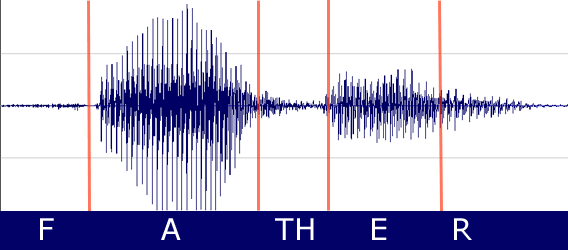Operating frequency choice

• A perfect human ear is sensible from 16 Hz to 22 kHz, but most of the formants have a frequency that is under 2300 - 2500 Hz, so it should be safe to sample at 11 kHz, thus enabling all the frequencies approximately up to 5 kHz to pass unbiased.

• The signal has been split in windows (or frames) having 100 samples of length, which corresponds to a length in time of 9.07 ms approximately.

• I will calculate the discrete Fourier Transform for every frame of the signal, for 20 different frequencies, corresponding to periods between 2 sampling periods and 100 sampling period.

• All the 20 frequencies have periods which are multiple of the sampling period, and

• they are as close as possible to a geometric progression as far as the previous constraint is respected.

This means that the minimum and maximum of the 20 frequencies at which the transform is calculated are :

 Maximum frequency: 5.5125 kHz Minimum frequency: 110.25 Hz

Fourier Transform

I have developed a stand-alone program (subsequently called FourierAnalysis), which calculates the Fourier Transform for a number of frequencies between the above minimum and maximum, giving at the same time a time-domain and a frequency-domain representation.

Its main two purposes are (training- and test-) pattern generation and data visualization.

The program is a 32-bit executable for Microsoft Windows, I wrote it in C++ with the support of the Microsoft Foundation Classes and compiled with the Microsoft Visual C++ compiler.

 The following example depicts the regular joint frequency- and time- domain representation representation of the waveform composing the english word 'father' provided by FourierAnalysis: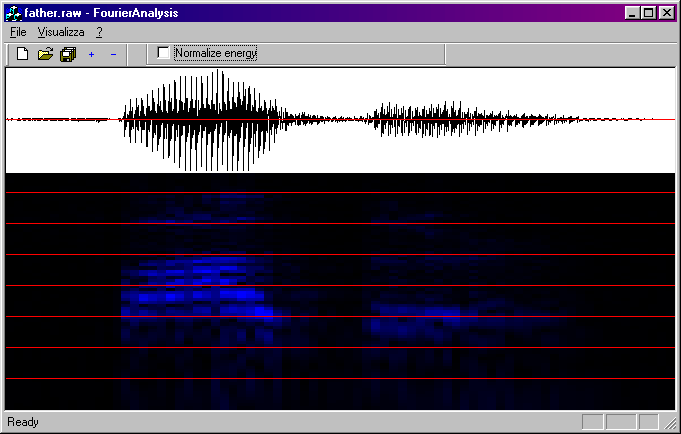Please note that in the 'regular' representation, all the spectral energies are represented on the same scale; therefore the power spectra of phonemes corresponding to the 'f', 'th' and 'r' consonants are practically invisible if represented on the same scale as the spectra of the vowels.

To avoid this problem, therefore allowing a good visualization of the consonant spectra, which contain much less energy with respect to their vowel counterparts, I introduced the possibility of a local normalization, which is computed for every window.

 The following picture depicts a joint representation provided by FourierAnalysis of the waveform composing the english word 'father' where every value has been normalized on a per-window basis: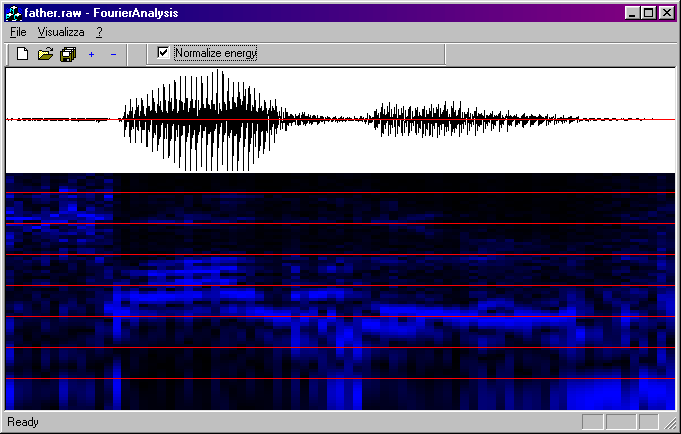The program exports at the same time:

• a compact-format data file, used by the neural network for training and recognition, containing the spectral energies at 20 frequencies as said above;

• an extended-format data file, which can be used by any other program, containing the spectral energies at 77 frequencies; the following picture shows an example of usage of this file with the Gnuplot application: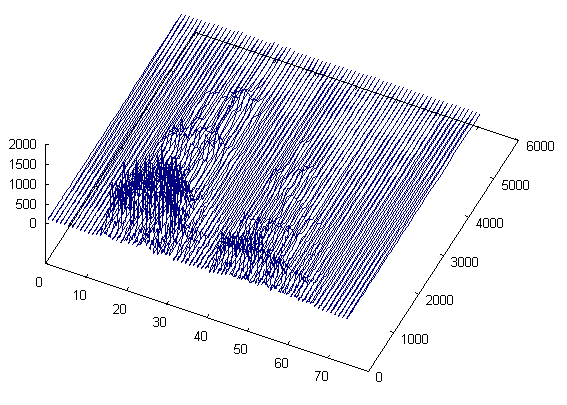Using a backpropagation network:

The first network I tried to employ for solving the recognition problem was the backpropagation network.

• easy error-evaluation

• very slow to train (minutes on a modern PC),

• training time complexity increases exponentially with number of pattern categories
(O(n) = 2n, basically due to the cardinality of the power set of the neuron set),

• inaccuracy if training process is not completed,

• suboptimal usage of the neurons,

• large number of neurons and layers required to improve results (?),

• unfair towards pattern categories with different cardinality;

Experiment details:

• the network is composed by two layers, containing 6 neurons each,

• each neuron takes as input 20 samples of the spectral energy diagram, at 20 different frequencies in geometric progression (their logarithms are equally spaced), as described above;

• the 6 neurons on the output layer are mapped to the 6 possible output phonemes,

• the network has been trained with 146 different patterns,

• divided in 6 categories (the vowels represented in the IPA form As /a/, /e/, /i:/, /o/, /u/ plus the sibilant consonant /s/),

• 30 iterations were performed (in each iteration each training patterns is submitted and the program tries to reduce the global error);

 The following picture shows the structure of the backpropagation network used. Each box represent a neuron composed of a set of summation weights and a non linear activation function.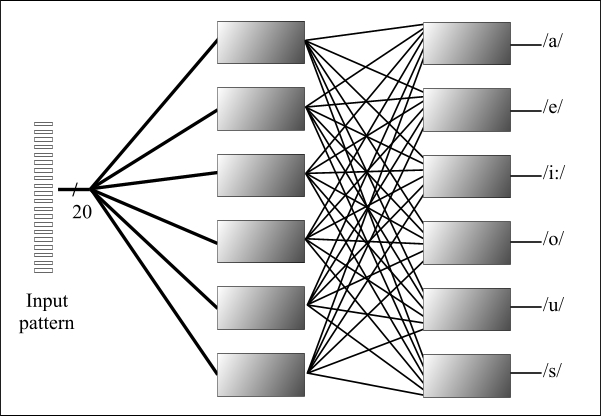Experiment result:
• after the training the network incorrectly recognizes a significant part (more than 30%) of the same patterns used for training;

• results are even worse when new test data is used for testing.

 The following graph depicts the behaviour of error with time. As you can see as time increases, it becomes more and more difficult to decrease the error. On the horizontal axis the current step number is represented (there is a step for each of the 146 input pattern in an iteration, there are 30 iterations). On the vertical axis the current value of the global error is represented; the global error is calculated as the sum of the local errors on all the patterns, where the local error for each pattern is the euclidean distance between the output vector and the desired output vector)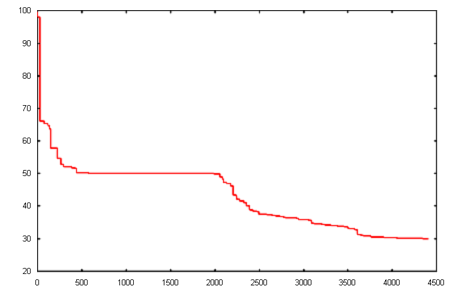Decision: I DISCARDED the backpropagation network in favour of another type of network, specifically designed for the purpose.

Using a Neuron Pool

Understanding the causes of the problems with the backpropagation network, I decided to use a modified network, with features coming from the Instar (the Kohonen Layer in the counterpropagation network) and from the LaMStAR architecture.

I will call this architecture neuron pool and it can be considered as a special case of a 1-layer LaMStAR network.

The neurons in a neuron pool are nothing more than distributed distance calculation nodes.

Features:

• Winner Takes All principle;

• one neuron for each recognized phoneme;

• deterministical training based on statistic properties of the input pattern

 The following graph depicts the structure of the neuron pool. Each node does not contain a weighed summation as in the backpropagation network, but a distance operator. This choice will be better explained later. There is no activation function.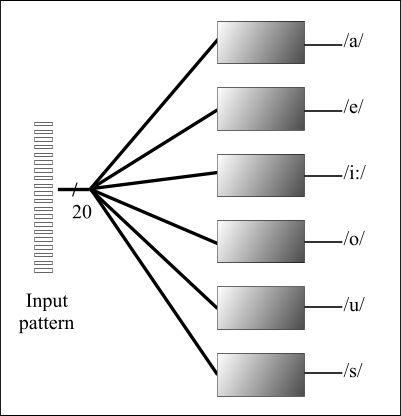• simple architecture (one layer only)

• extremely fast training (O(n) = n, linear with the number of training patterns),

• optimal use of the neurons (one for every phoneme),

• small number of neurons required,

• if you find someone let me know.

Testing patterns:
 The following picture depicts the joint time-domain and frequency domain-representation of the patterns used to test the network: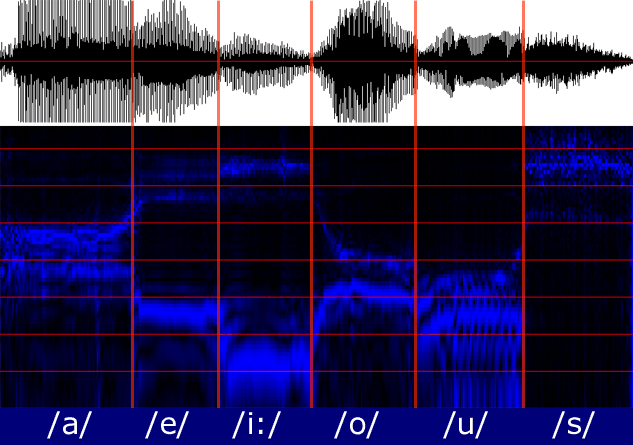Neuron Pool: data preprocessing

 Goal: the recognition of a phoneme should be independent from the signal power; the same phoneme should be recognized if the speaker is talking loud or weakly; To achieve this goal we normalize the input pattern, by performing the following actions: calculating the average of the values in the pattern; subtracting to each value the average; calculating the variance on the new pattern; dividing every value by the square root of the variance; This normalization has also other interesting advantages: keeping the values in a well-defined field (immediately below the unity) guarantees numerical accuracy preventing loss of significant digits; allows us to use the distance algorithm, better explained below;

Neuron Pool: training algorithm

 The following algorithm has been used: for every recognized phoneme: create a new neuron for every weight calculate the average of the values for the frequency corresponding to that weight in the normalized training patterns; set the weight value to that average;

Neuron Pool: who is the winner ?   or
'An /i:/ is not a /u/'

The result of the recognition process is the phoneme associated to the winning neuron.

Problem: which of the winning neuron should be declared the winner ?

 Notation: - - - - - - -- - - - - - - - - - -- - - - - - - - - - -- - - - - - - - - - -- - - - - - - - - - - - - - - - - - - - - - - - - - - -- - - - - - - x is the input pattern vector, its elements are called x1, x2, ... wi is the weight vector for the i-th neuron, its elements are called wi,1, wi,2, ...

 Dot product - - - - - - - - - -- - - - - - - - - - - - - - - - - - - - - - - - - - - -- - - - - - - zi = x . wi = Sj xj . wi,j The winner i is the one with the maximum zi. Distance - - - - - - -- - - - - - - - - - -- - - - - - - - - - -- - - - - - - - - - -- - - - - - - - - di = || x - wi || = ( Sj ( xj - wi,j ) 2 ) 1/2 The winner i is the one with the minimum di.

Note: what I'm going to say still holds even if an activation function is applied to the distance or to the dot product as soon as the monothonical increasing function hypothesis holds.

Our experiments show that the distance method is much more accurate than the dot product, and after changing from the dot product to the distance method the results have improved.

The following table reports the number of training patterns used for every phoneme:

 Phoneme number of training patterns /a/ 236 /e/ 196 /i/ 131 /o/ 205 /u/ 477 /s/ 29 total 1274

The following table reports the error rate of the two methods when testing the same pattern set used for training:

Dot product - - - - - - - - - -- - - - - - - - - - - - - - - - - - - - - - - - - - - -- - - - - - -
 Errors: 119 Error rate: 9.341 %
Distance - - - - - - -- - - - - - - - - - -- - - - - - - - - - -- - - - - - - - - - -- - - - - - - - -
 Errors: 24 Error rate: 1.884 %

If we better analyze the errors in the dot product we discover that most of them are due to a /i:/ phoneme recognized as a /u/. This is a good example to show that the dot product is less accurate than the distance method for this application: in this case the dot product of some /i:/ input patterns' values and the optimal weights for the (wrong) /u/ neuron is greater than the dot product of the same input values and the optimal weights for the (right) /i:/ neuron.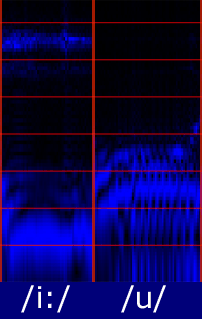It is easy to visually explain what happened by comparing the spectra of the /i:/ and of the /u/ phonemes: The spectum energy for the /i:/ is composed by two formants. one of which is at low frequency (250 Hz approx) and the second of which is at high frequency (2.2 kHz approx) while the /u/ has all the formants between 200 Hz and 1 kHz. It is therefore possible, applying the /u/ recognition neuron over a /i:/ pattern slightly raised in frequency, to (incorrectly) achieve a resulting dot product that is greater than the dot product obtained applying the (right) /i:/ recognition neuron, due to the fact that what is lost by negliging the high frequency formant of the /i:/, is gained by capturing the tail of the /i:/ low frequency formant in the 0.5-1.0 kHz frequency range. This problem is avoided by using the distance method, which 'punishes' the /u/ neuron twice: for not taking into account the /i:/ high frequency formant and for expecting a more extended low freuency formant.

Improvement: a reliability indicator

Let me introduce the following indicator: the winning ratio.

I define the winning ratio as the ratio between the distance from the input pattern and the best matching neuron and the distance from the input pattern and the second-best matching neuron. More formally:

 Winning ratio - - - - - - - - - -- - - - - - - - - - - - - - - - -- - - - - - - - - - - - - - - - -- - - - - - - - - - - - - - - - -- - - - - - - - - - - - - - - - - - - - - - - - - - - -- - - - - - - r = || x - wi1 || / || x - wi2 || where: x is the input pattern vector, wi is the weight vector for the i-th neuron, i1 is the index of the best matching neuron (the one with the minimum distance), i2 is the index of the second best matching neuron.

Intuitively:

• A low winning ratio (e.g. 0.01 - 0.1) indicates that the best neuron matches the input pattern much better than any other neuron.

• A high winning ratio (e.g. 0.5 - 0.9) indicates that there is at least one other neuron which matches pretty well the input pattern, i.e. its distance from the pattern is similar (2x - 1.11x) to the best neuron's one.

For short:

• a low winning ratio means low recognition uncertainty;

• a high winning ratio means high recognition uncertainty;

 Experimental observation The following picture depicts a part of the recognition log for the /e/ phoneme. It is easy to see that upon error (errors are marked with a red arrow) the winning ratio is high (0.6-0.9) while in the other cases the winning ratio is low (less than 0.1).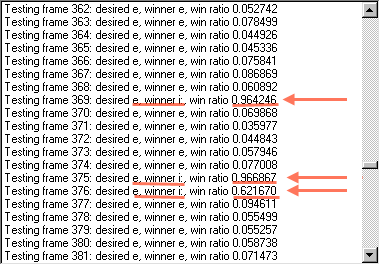It should be now a quite reasonable solution -provided that there is a sufficiently large number of frames containing the same phoneme to recognize- to discard the recognition results which have a degree of uncertainty (winning ratio) greater than a suitable threshold.

I will not discuss which is the optimal value for this threshold, I only want to report the results of introducing some sample thresholds in the recognition process; all the other conditions in the experiment are the same as the previous experiment with the 'distance' method (same training patterns, same algorithm).

It is easily understood how lowering the threshold too much yields a large number of killings also in the correctly recognized frames.

Results

 Threshold value: 0.4 0.5 0.6 (no threshold) Errors: 7 8 13 24 Error rate: 0.549 % 0.628 % 1.020 % 1.884 % Wrong killed frames: 17 16 11 0 Correct killed frames: 124 65 35 0

Improvement: killing lonely phonemes

The last phase of the recognition is the translation from the sequence of winner neurons: (here a phoneme is repeated as many times as the number of frames in which the neuron associated to that phoneme was the winner)

 /a/ /a/ /a/ /a/ /a/ /a/ /a/ /a/ /a/ /a/ /a/ /a/ /a/ /a/ /a/ /a/ /a/ /a/ /a/ /a/ /a/ /a/ /a/ /a/ /a/ /a/ /a/ /a/ /a/ /a/ /a/ /a/ /a/ /a/ /a/ /a/ /a/ /a/ /a/ /a/ /a/ /a/ /a/ /a/ /a/ /a/ /a/ /a/ /a/ /a/ /a/ /a/ /a/ /a/ /a/ /a/ /a/ /a/ /a/ /a/ /a/ /a/ /a/ /a/ /a/ /a/ /a/ /a/ /a/ /a/ /a/ /a/ /a/ /a/ /a/ /a/ /a/ /a/ /a/ /a/ /a/ /a/ /a/ /a/ /a/ /a/ /a/ /a/ /a/ /a/ /a/ /a/ /a/ /a/ /a/ /a/ /a/ /a/ /a/ /a/ /a/ /a/ /a/ /a/ /a/ /a/ /a/ /a/ /a/ /a/ /a/ /a/ /a/ /a/ /a/ /a/ /a/ /a/ /a/ /a/ /a/ /a/ /a/ /a/ /a/ /a/ /a/ /a/ /a/ /a/ /a/ /a/ /a/ /a/ /a/ /a/ /a/ /a/ /a/ /a/ /a/ /a/ /a/ /a/ /a/ /a/ /a/ /a/ /a/ /a/ /a/ /a/ /a/ /a/ /a/ /a/ /a/ /a/ /a/ /a/ /a/ /a/ /a/ /a/ /a/ /a/ /a/ /a/ /a/ /a/ /a/ /a/ /a/ /a/ /a/ /a/ /a/ /a/ /a/ /a/ /a/ /a/ /a/ /a/ /a/ /a/ /a/ /a/ /a/ /a/ /a/ /a/ /a/ /a/ /a/ /a/ /a/ /a/ /a/ /a/ /a/ /a/ /a/ /a/ /a/ /a/ /a/ /a/ /a/ /a/ /a/ /a/ /a/ /a/ /a/ /a/ /a/ /a/ /a/ /a/ /a/ /a/ /a/ /a/ /a/ /a/ /a/ /a/ /a/ /a/ /a/ /a/ /a/ /a/ /a/ /a/ /e/ /e/ /e/ /e/ /e/ /e/ /e/ /e/ /e/ /e/ /e/ /e/ /e/ /e/ /e/ /e/ /e/ /e/ /e/ /e/ /e/ /e/ /e/ /e/ /e/ /e/ /e/ /e/ /e/ /e/ /e/ /e/ /e/ /e/ /e/ /e/ /e/ /e/ /e/ /e/ /e/ /e/ /e/ /e/ /e/ /e/ /e/ /e/ /e/ /e/ /e/ /e/ /e/ /e/ /e/ /e/ /e/ /e/ /e/ /e/ /e/ /e/ /e/ /e/ /e/ /e/ /e/ /e/ /e/ /e/ /e/ /e/ /e/ /e/ /e/ /e/ /e/ /e/ /e/ /e/ /e/ /e/ /e/ /e/ /e/ /e/ /e/ /e/ /e/ /e/ /e/ /e/ /e/ /e/ /e/ /e/ /e/ /e/ /e/ /e/ /e/ /e/ /e/ /e/ /e/ /e/ /e/ /e/ /e/ /e/ /e/ /e/ /e/ /e/ /e/ /e/ /e/ /e/ /e/ /e/ /e/ /e/ /e/ /e/ /e/ /e/ /e/ /e/ /e/ /e/ /e/ /e/ /e/ /e/ /e/ /e/ /e/ /e/ /e/ /e/ /e/ /e/ /e/ /e/ /e/ /e/ /e/ /e/ /e/ /e/ /e/ /e/ /e/ /e/ /e/ /e/ /e/ /e/ /e/ /e/ /e/ /e/ /e/ /e/ /e/ /e/ /i:/ /e/ /e/ /e/ /e/ /e/ /e/ /e/ /i:/ /e/ /e/ /e/ /e/ /e/ /e/ /e/ /e/ /i:/ /i:/ /i:/ /i:/ /i:/ /i:/ /i:/ /i:/ /i:/ /i:/ /i:/ /i:/ /i:/ /i:/ /i:/ /i:/ /i:/ /i:/ /i:/ /i:/ /i:/ /i:/ /i:/ /i:/ /i:/ /i:/ /i:/ /i:/ /i:/ /i:/ /i:/ /i:/ /i:/ /i:/ /i:/ /i:/ /i:/ /i:/ /i:/ /i:/ /i:/ /i:/ /i:/ /i:/ /i:/ /i:/ /i:/ /i:/ /i:/ /i:/ /i:/ /i:/ /i:/ /u/ /e/ /i:/ /i:/ /i:/ /i:/ /i:/ /i:/ /i:/ /i:/ /i:/ /e/ /i:/ /i:/ /i:/ /i:/ /i:/ /i:/ /e/ /o/ /o/ /o/ /o/ /o/ /o/ /o/ /o/ /o/ /o/ /o/ /o/ /o/ /o/ /o/ /o/ /o/ /o/ /o/ /o/ /o/ /o/ /o/ /o/ /o/ /o/ /o/ /o/ /o/ /o/ /o/ /o/ /o/ /o/ /o/ /o/ /o/ /o/ /o/ /o/ /o/ /o/ /o/ /o/ /o/ /o/ /o/ /o/ /o/ /o/ /o/ /o/ /o/ /o/ /o/ /o/ /o/ /o/ /o/ /o/ /o/ /o/ /o/ /o/ /o/ /o/ /o/ /o/ /o/ /o/ /o/ /o/ /o/ /o/ /o/ /o/ /o/ /o/ /o/ /o/ /o/ /o/ /o/ /o/ /o/ /o/ /o/ /o/ /o/ /o/ /o/ /o/ /o/ /o/ /o/ /o/ /o/ /o/ /o/ /o/ /o/ /o/ /o/ /o/ /o/ /o/ /o/ /o/ /o/ /o/ /o/ /o/ /o/ /o/ /o/ /o/ /o/ /o/ /o/ /o/ /o/ /o/ /o/ /o/ /o/ /o/ /o/ /o/ /o/ /o/ /o/ /o/ /o/ /o/ /o/ /o/ /o/ /o/ /o/ /o/ /o/ /o/ /o/ /o/ /o/ /o/ /o/ /o/ /o/ /o/ /o/ /o/ /o/ /o/ /o/ /o/ /o/ /o/ /o/ /o/ /o/ /o/ /o/ /o/ /o/ /o/ /o/ /o/ /o/ /o/ /o/ /o/ /o/ /o/ /o/ /o/ /o/ /o/ /o/ /o/ /o/ /o/ /o/ /o/ /o/ /o/ /o/ /o/ /o/ /o/ /o/ /o/ /o/ /o/ /o/ /o/ /o/ /o/ /o/ /o/ /o/ /u/ /u/ /u/ /u/ /u/ /u/ /u/ /u/ /u/ /u/ /u/ /u/ /u/ /u/ /u/ /u/ /u/ /u/ /u/ /u/ /u/ /u/ /u/ /u/ /u/ /u/ /u/ /u/ /u/ /u/ /u/ /u/ /u/ /u/ /u/ /u/ /u/ /u/ /u/ /u/ /u/ /u/ /u/ /u/ /u/ /u/ /u/ /u/ /u/ /u/ /u/ /u/ /u/ /u/ /u/ /u/ /u/ /u/ /u/ /u/ /u/ /u/ /u/ /u/ /u/ /u/ /u/ /u/ /u/ /u/ /u/ /u/ /u/ /u/ /u/ /u/ /u/ /u/ /u/ /u/ /u/ /u/ /u/ /u/ /u/ /u/ /u/ /u/ /u/ /u/ /u/ /u/ /u/ /u/ /u/ /u/ /u/ /u/ /u/ /u/ /u/ /u/ /u/ /u/ /u/ /u/ /u/ /u/ /u/ /u/ /u/ /u/ /u/ /u/ /u/ /u/ /u/ /u/ /u/ /u/ /u/ /u/ /u/ /u/ /u/ /u/ /u/ /u/ /u/ /u/ /u/ /u/ /u/ /u/ /u/ /u/ /u/ /u/ /u/ /u/ /u/ /u/ /u/ /u/ /u/ /u/ /u/ /u/ /u/ /u/ /u/ /u/ /u/ /u/ /u/ /u/ /u/ /u/ /u/ /u/ /u/ /u/ /u/ /u/ /u/ /u/ /u/ /u/ /u/ /u/ /u/ /u/ /u/ /u/ /u/ /u/ /u/ /u/ /u/ /u/ /u/ /u/ /u/ /u/ /u/ /u/ /u/ /u/ /u/ /u/ /u/ /u/ /u/ /u/ /u/ /u/ /u/ /u/ /u/ /u/ /u/ /u/ /u/ /u/ /u/ /u/ /u/ /u/ /u/ /u/ /u/ /u/ /u/ /u/ /u/ /u/ /u/ /u/ /u/ /u/ /u/ /u/ /u/ /u/ /u/ /u/ /u/ /u/ /u/ /u/ /u/ /u/ /u/ /u/ /u/ /u/ /u/ /u/ /u/ /u/ /u/ /u/ /u/ /u/ /u/ /u/ /u/ /u/ /u/ /u/ /u/ /u/ /u/ /u/ /u/ /u/ /u/ /u/ /u/ /u/ /u/ /u/ /u/ /u/ /u/ /u/ /u/ /u/ /u/ /u/ /u/ /u/ /u/ /u/ /u/ /u/ /u/ /u/ /u/ /u/ /u/ /u/ /u/ /u/ /u/ /u/ /u/ /u/ /u/ /u/ /u/ /u/ /u/ /u/ /u/ /u/ /u/ /u/ /u/ /u/ /u/ /u/ /u/ /u/ /u/ /u/ /u/ /u/ /u/ /u/ /u/ /u/ /u/ /u/ /u/ /u/ /u/ /u/ /u/ /u/ /u/ /u/ /u/ /u/ /u/ /u/ /u/ /u/ /u/ /u/ /u/ /u/ /u/ /u/ /u/ /u/ /u/ /u/ /u/ /u/ /u/ /u/ /u/ /u/ /u/ /u/ /u/ /u/ /u/ /u/ /u/ /u/ /u/ /u/ /u/ /u/ /u/ /u/ /u/ /u/ /u/ /u/ /u/ /u/ /u/ /u/ /u/ /u/ /u/ /u/ /u/ /u/ /u/ /u/ /u/ /u/ /u/ /u/ /u/ /u/ /u/ /u/ /u/ /u/ /u/ /u/ /u/ /u/ /u/ /u/ /u/ /u/ /u/ /u/ /u/ /u/ /u/ /u/ /u/ /u/ /u/ /u/ /u/ /u/ /u/ /u/ /u/ /u/ /u/ /u/ /u/ /u/ /u/ /u/ /u/ /u/ /u/ /u/ /u/ /u/ /u/ /u/ /u/ /u/ /u/ /u/ /u/ /u/ /u/ /u/ /u/ /u/ /u/ /u/ /u/ /u/ /u/ /u/ /u/ /u/ /u/ /u/ /u/ /u/ /u/ /u/ /u/ /u/ /u/ /u/ /u/ /u/ /u/ /u/ /u/ /u/ /u/ /u/ /u/ /u/ /u/ /u/ /u/ /u/ /u/ /u/ /u/ /u/ /u/ /u/ /u/ /u/ /u/ /s/ /s/ /s/ /s/ /s/ /s/ /s/ /s/ /s/ /s/ /s/ /s/ /s/ /s/ /s/ /s/ /s/ /s/ /s/ /s/ /s/ /s/ /s/ /s/ /s/

to the final phonetic transcription of the recognized word:

 /a/ /e/ /i:/ /o/ /u/ /s/

(here the phoneme appears according to the phonetic of the word)

Let's now introduce an intermediate representation, obtained by reading the sequence of winners, counting the repetitions for each phoneme and replacing them with a (phoneme, repetition count) couple:

 (/a/, 236) (/e/, 166) (/i:/, 1) (/e/, 7) (/i:/, 1) (/e/, 8) (/i:/, 53) (/u/, 1) (/e/, 1) (/i:/, 9) (/e/, 1) (/i:/, 6) (/e/, 3) (/o/, 202) (/u/, 473) (/s/, 25)

Experimental evidences show that errors in the recognition phase appear as short sequences of phonemes with a low repetition count. It seems now reasonable to tag as errors and remove all the sequences of phonemes with a repetition count less than a suitable threshold.

In this case I used the value 5 as threshold, and these are the new results, after removing the short sequences and packing together the rest:

 (/a/, 236) (/e/, 181) (/i:/, 68) (/o/, 202) (/u/, 473) (/s/, 25)

Obtaining the final phonetic transcription from this representation is trivial.

Examples and results:

The results we will report were produced under the following conditions:
• loneliness killing threshold: 4 repetitions

• winning ratio threshold: 0.8

• training patterns as below:

 Phoneme number of training patterns /a/ 236 /e/ 171 /i/ 104 /o/ 205 /u/ 169 /s/ 29 /m/ 78 total 992

Training details: the log of the training is here reported. The omitted parts contain no errors and no interesting data.
```Testing frame 0: desired /a/, winner /a/, win ratio 0.000000
Testing frame 1: desired /a/, winner /a/, win ratio 0.000000
Testing frame 2: desired /a/, winner /a/, win ratio 0.000000
...
Testing frame 234: desired /a/, winner /a/, win ratio 0.000000
Testing frame 235: desired /a/, winner /a/, win ratio 0.000000
Testing frame 236: desired /e/, winner /e/, win ratio 0.103249
Testing frame 237: desired /e/, winner /e/, win ratio 0.101542
...
Testing frame 405: desired /e/, winner /e/, win ratio 0.050582
Testing frame 406: desired /e/, winner /e/, win ratio 0.079193
Testing frame 407: desired /i:/, winner /i:/, win ratio 0.275368
Testing frame 408: desired /i:/, winner /i:/, win ratio 0.279233
Testing frame 409: desired /i:/, winner /m/, win ratio 0.604378
Testing frame 410: desired /i:/, winner /i:/, win ratio 0.402855
Testing frame 411: desired /i:/, winner /i:/, win ratio 0.216688
...
Testing frame 509: desired /i:/, winner /i:/, win ratio 0.059101
Testing frame 510: desired /i:/, winner /i:/, win ratio 0.047175
Testing frame 511: desired /o/, winner /o/, win ratio 0.399943
Testing frame 512: desired /o/, winner /o/, win ratio 0.186259
...
Testing frame 713: desired /o/, winner /o/, win ratio 0.194598
Testing frame 714: desired /o/, winner /o/, win ratio 0.295468
Testing frame 715: desired /o/, winner /m/, win ratio 0.805574
Testing frame 716: desired /u/, winner /u/, win ratio 0.230878
Testing frame 717: desired /u/, winner /u/, win ratio 0.188211
...
Testing frame 883: desired /u/, winner /u/, win ratio 0.121368
Testing frame 884: desired /u/, winner /u/, win ratio 0.429669
Testing frame 885: desired /s/, winner /m/, win ratio 0.826323
Testing frame 886: desired /s/, winner /s/, win ratio 0.695413
Testing frame 887: desired /s/, winner /i:/, win ratio 0.203407
Testing frame 888: desired /s/, winner /s/, win ratio 0.218484
Testing frame 889: desired /s/, winner /s/, win ratio 0.206388
...
Testing frame 912: desired /s/, winner /s/, win ratio 0.590790
Testing frame 913: desired /s/, winner /s/, win ratio 0.489405
Testing frame 914: desired /m/, winner /o/, win ratio 0.979688
Testing frame 915: desired /m/, winner /o/, win ratio 0.527820
Testing frame 916: desired /m/, winner /m/, win ratio 0.787123
Testing frame 917: desired /m/, winner /m/, win ratio 0.860028
...
Testing frame 990: desired /m/, winner /m/, win ratio 0.218466
Testing frame 991: desired /m/, winner /m/, win ratio 0.160790
Errors = 4, error percentage = 0.403 %
Recognized string postprocessing:  /a/ /a/ /a/ /a/ /a/ /a/ /a/ /a/ /a/
...
/a/ /a/ /a/ /a/ /a/ /a/ /a/ /a/ /a/ /a/ /a/ /a/ /a/ /a/ /a/ /a/ /a/
/a/ /a/ /a/ /a/ /a/ /a/ /e/ /e/ /e/ /e/ /e/ /e/ /e/ /e/ /e/ /e/ /e/
/e/ /e/ /e/ /e/ /e/ /e/ /e/ /e/ /e/ /e/ /e/ /e/ /e/ /e/ /e/ /e/ /e/
/e/ /e/ /e/ /e/ /e/ /e/ /e/ /e/ /e/ /e/ /e/ /e/ /e/ /e/ /e/ /e/ /e/
...
/e/ /e/ /e/ /e/ /e/ /e/ /e/ /e/ /e/ /e/ /e/ /e/ /e/ /e/ /e/ /e/ /e/
/e/ /e/ /e/ /e/ /e/ /e/ /e/ /i:/ /i:/ /m/ /i:/ /i:/ /i:/ /i:/ /i:/
/i:/ /i:/ /i:/ /i:/ /i:/ /i:/ /i:/ /i:/ /i:/ /i:/ /i:/ /i:/ /i:/ /i:/
...
/i:/ /i:/ /i:/ /i:/ /i:/ /i:/ /i:/ /i:/ /i:/ /i:/ /i:/ /i:/ /i:/ /i:/
/i:/ /i:/ /i:/ /i:/ /i:/ /i:/ /i:/ /i:/ /i:/ /i:/ /i:/ /i:/ /o/ /o/ /o/
/o/ /o/ /o/ /o/ /o/ /o/ /o/ /o/ /o/ /o/ /o/ /o/ /o/ /o/ /o/ /o/ /o/ /o/
...
/o/ /o/ /o/ /o/ /o/ /o/ /o/ /o/ /o/ /o/ /o/ /o/ /o/ /o/ /o/ /o/ /o/ /o/
/o/ /o/ /o/ /u/ /u/ /u/ /u/ /u/ /u/ /u/ /u/ /u/ /u/ /u/ /u/ /u/ /u/ /u/
/u/ /u/ /u/ /u/ /u/ /u/ /u/ /u/ /u/ /u/ /u/ /u/ /u/ /u/ /u/ /u/ /u/ /u/
...
/u/ /u/ /u/ /u/ /u/ /u/ /u/ /u/ /u/ /u/ /u/ /u/ /u/ /u/ /u/ /u/ /u/ /u/
/u/ /u/ /u/ /u/ /u/ /u/ /u/ /u/ /u/ /u/ /s/ /i:/ /s/ /s/ /s/ /s/ /s/ /s/
/s/ /s/ /s/ /s/ /s/ /s/ /s/ /s/ /s/ /s/ /s/ /s/ /s/ /s/ /s/ /s/ /s/ /s/
/s/ /s/ /o/ /m/ /m/ /m/ /m/ /m/ /m/ /m/ /u/ /m/ /m/ /m/ /m/ /m/ /m/ /m/
/m/ /m/ /m/ /m/ /m/ /m/ /m/ /m/ /m/ /m/ /m/ /m/ /m/ /m/ /m/ /m/ /m/ /m/
...
/m/ /m/ /m/ /m/ /m/ /m/ /m/ /m/ /m/ /m/ /m/ /m/ /m/ /m/ /m/ /m/ /m/ /m/
/m/ /m/ /m/ /m/
After encoding change: (/a/, 236) (/e/, 171) (/i:/, 2) (/m/, 1) (/i:/, 101)
(/o/, 204) (/u/, 169) (/s/, 1) (/i:/, 1) (/s/, 26) (/o/, 1) (/m/, 7) (/u/, 1) (/m/, 65)
Average repetition value = 70.428571
Repetition value variance = 5.030612
After removing and soldering:
(/a/, 236) (/e/, 171) (/i:/, 101) (/o/, 204) (/u/, 169) (/s/, 26) (/m/, 72)
After killing lonely phonemes: /a/ /e/ /i:/ /o/ /u/ /s/ /m/
```

Results: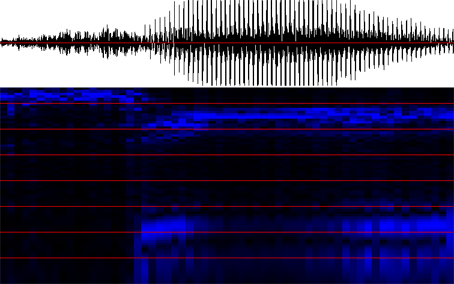"see" (English) [si:] Recognized string postprocessing: /s/ /s/ /s/ /s/ /s/ /s/ /s/ /s/ /s/ /s/ /s/ /s/ /s/ /s/ /s/ /s/ /s/ /s/ /i:/ /e/ /u/ /i:/ /s/ /i:/ /i:/ /i:/ /i:/ /i:/ /i:/ /i:/ /i:/ /i:/ /i:/ /i:/ /i:/ /i:/ /i:/ /i:/ /i:/ /i:/ /i:/ /i:/ /i:/ /i:/ /i:/ /i:/ /i:/ /m/ /i:/ /i:/ /i:/ /i:/ /m/ After encoding change: (/s/, 18) (/i:/, 1) (/e/, 1) (/u/, 1) (/i:/, 1) (/s/, 1) (/i:/, 24) (/m/, 1) (/i:/, 4) (/m/, 1) Average repetition value = 5.300000 Repetition value variance = 0.530000 After removing and soldering: (/s/, 18) (/i:/, 28) After killing lonely phonemes: /s/ /i:/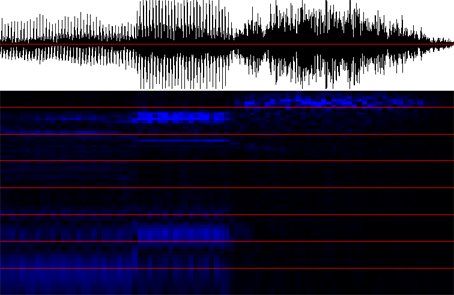"miss" (English) [mi:s] Recognized string postprocessing: /m/ /m/ /m/ /m/ /m/ /m/ /m/ /m/ /m/ /m/ /m/ /m/ /m/ /m/ /m/ /m/ /m/ /m/ /m/ /m/ /m/ /m/ /m/ /m/ /m/ /m/ /m/ /i:/ /i:/ /i:/ /i:/ /i:/ /i:/ /i:/ /i:/ /i:/ /i:/ /i:/ /i:/ /i:/ /i:/ /i:/ /i:/ /i:/ /i:/ /i:/ /s/ /s/ /s/ /s/ /s/ /s/ /s/ /s/ /s/ /s/ /s/ /s/ /s/ /s/ /s/ /s/ /s/ /s/ /s/ /s/ /s/ /s/ /s/ /s/ /s/ /s/ /s/ /s/ /s/ /s/ /s/ /s/ /s/ /s/ /s/ /s/ /s/ /s/ /s/ /s/ /s/ /s/ /s/ After encoding change: (/m/, 27) (/i:/, 19) (/s/, 43) Average repetition value = 29.666667 Repetition value variance = 9.888889 After removing and soldering: (/m/, 27) (/i:/, 19) (/s/, 43) After killing lonely phonemes: /m/ /i:/ /s/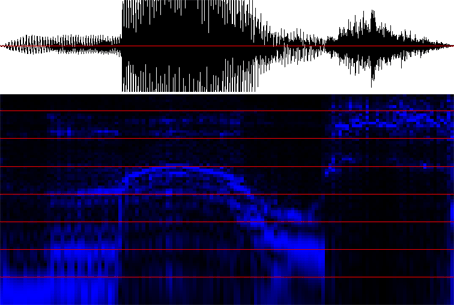"maus" (German) [maus] Recognized string postprocessing: /m/ /m/ /m/ /m/ /m/ /m/ /m/ /m/ /m/ /m/ /m/ /m/ /m/ /m/ /m/ /m/ /m/ /m/ /m/ /m/ /m/ /m/ /m/ /a/ /a/ /a/ /a/ /a/ /a/ /a/ /a/ /a/ /a/ /a/ /a/ /a/ /a/ /a/ /a/ /a/ /a/ /a/ /a/ /a/ /a/ /a/ /a/ /a/ /a/ /a/ /a/ /a/ /a/ /a/ /a/ /a/ /a/ /o/ /o/ /o/ /u/ /e/ /m/ /u/ /u/ /u/ /u/ /u/ /u/ /u/ /u/ /u/ /m/ /s/ /s/ /s/ /s/ /s/ /s/ /s/ /s/ /s/ /s/ /s/ /s/ /s/ /s/ /s/ /s/ /s/ /s/ /s/ /s/ /s/ /s/ /s/ /s/ /s/ /s/ /s/ /s/ /s/ /s/ /s/ /s/ /s/ /s/ /s/ /s/ /s/ /o/ After encoding change: (/m/, 23) (/a/, 34) (/o/, 3) (/u/, 1) (/e/, 1) (/m/, 1) (/u/, 9) (/m/, 1) (/s/, 37) (/o/, 1) Average repetition value = 11.100000 Repetition value variance = 1.110000 After removing and soldering: (/m/, 23) (/a/, 34) (/u/, 9) (/s/, 37) After killing lonely phonemes: /m/ /a/ /u/ /s/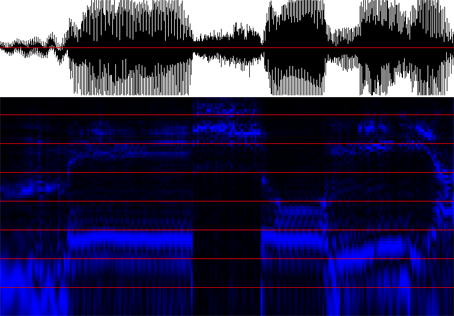"mé so měa" (Dialect Cremasco) [me so 'mi:a] Recognized string postprocessing: /m/ /m/ /m/ /m/ /m/ /m/ /m/ /m/ /m/ /m/ /m/ /m/ /m/ /m/ /m/ /m/ /m/ /m/ /m/ /m/ /m/ /m/ /m/ /m/ /m/ /m/ /m/ /m/ /m/ /m/ /e/ /e/ /e/ /e/ /e/ /e/ /e/ /e/ /e/ /e/ /e/ /e/ /e/ /e/ /e/ /e/ /e/ /e/ /e/ /e/ /e/ /e/ /e/ /e/ /e/ /e/ /e/ /e/ /e/ /e/ /e/ /e/ /e/ /e/ /e/ /e/ /e/ /e/ /e/ /e/ /e/ /e/ /e/ /e/ /e/ /e/ /e/ /e/ /e/ /e/ /e/ /e/ /e/ /e/ /e/ /e/ /e/ /e/ /e/ /m/ /s/ /s/ /s/ /s/ /s/ /s/ /s/ /s/ /s/ /s/ /s/ /s/ /s/ /s/ /s/ /s/ /s/ /s/ /s/ /s/ /s/ /s/ /s/ /s/ /s/ /s/ /s/ /s/ /s/ /s/ /s/ /s/ /s/ /s/ /m/ /e/ /o/ /o/ /o/ /o/ /o/ /o/ /o/ /o/ /o/ /o/ /o/ /o/ /o/ /o/ /o/ /o/ /o/ /o/ /a/ /o/ /m/ /m/ /m/ /m/ /u/ /m/ /m/ /m/ /u/ /u/ /i:/ /u/ /i:/ /i:/ /i:/ /i:/ /i:/ /e/ /m/ /m/ /e/ /m/ /m/ /m/ /e/ /m/ /m/ /m/ /m/ /m/ /m/ /m/ /m/ /m/ /u/ /u/ /u/ /s/ /e/ /e/ /e/ /a/ /a/ /a/ /a/ /a/ /a/ /a/ /a/ /a/ After encoding change: (/m/, 30) (/e/, 59) (/m/, 1) (/s/, 34) (/m/, 1) (/e/, 1) (/o/, 18) (/a/, 1) (/o/, 1) (/m/, 4) (/u/, 1) (/m/, 3) (/u/, 2) (/i:/, 1) (/u/, 1) (/i:/, 5) (/e/, 1) (/m/, 2) (/e/, 1) (/m/, 3) (/e/, 1) (/m/, 9) (/u/, 3) (/s/, 1) (/e/, 3) (/a/, 9) Average repetition value = 7.538462 Repetition value variance = 0.289941 After removing and soldering: (/m/, 30) (/e/, 59) (/s/, 34) (/o/, 18) (/m/, 4) (/i:/, 5) (/m/, 9) (/a/, 9) After killing lonely phonemes: /m/ /e/ /s/ /o/ /m/ /i:/ /m/ /a/

Result evaluation:

 My experiments show that the network architecture reaches a pretty good degree of accuracy in recognizing the voice of a speaker, as far as the phonemes in the testing phase are not pronounced too much differently from the ones used in the training phase. Limitations and possible future improvements: the network so far is speaker-dependent; extending its capabilities in order to recognize the voices of multiple speakers (or, even better, of any possible speaker by usign some sort of generalization process) would not be a trivial task, but a good percentage of this work should still keep its validity; one of the network's weaknesses is the recognition of transitional sounds between one phoneme and the other; in my work this problem was treated with an easy (but not accurate) solution: since transitions are short in time, simply ignoring the strange recognition results represented by short sequences should be enough. A better architecture could be trained not only on phonemes but also on transitions, in order take advantage of transitional sounds to improve the recognition reliability instead of trashing them; the network could be improved by adding a cascading additional module which is trained to know a dictionary and a probabilistic model of phoneme sequences, which takes as input the intermediate recognition representation as introduced above, and: performs the 'lonely phoneme killings' in a smarter way, using probabilistic principles such as the most likelihood; translates the final phonetic representation in a written language representation, possibly resolving ambiguities between words with the same pronounce; ...

Bibliography:

 Hosom, Cole, Fanty, Scahlkwyk, Yan, Wei, Training Neural networks for Speech Recognition, Centre for Spoken Language Understanding, Oregon Graduate Institute of Science and Tecnology, 1999 Scahlkwyk, Hosom, Kaiser, Shobaki The CSLU Hidden Markov Modeling Environment, Centre for Spoken Language Understanding, Oregon Graduate Institute of Science and Tecnology, 2000 Online resources: cslu.cse.ogi.edu, Centre for Spoken Language Understanding, Oregon Graduate Institute of Science and Tecnology Hieronymus, ASCII Phonetic Symbols for the World's Languages: Worldbet, AT&T Bell Laboratories

110 184 323 569 1000 1759 3092 5437